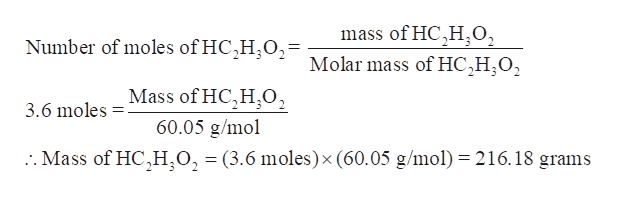# How many grams of HC2H3O2 are there in 3.6 moles of HC2H3O2?

Question

How many grams of HC2H3O2 are there in 3.6 moles of HC2H3O2?

check_circleExpert Solution
Step 1

The Mass (in grams) of HC2H3O2 in 3.6 moles of HC2H3O2 is to be calculated.

Step 2

The number of moles of a substance is defined as the given mass of the substance divided by the molar mass of that substance.

Step 3

Now, calculate the mass of HC2H3O2 in 3.6 moles-

Moles of HC2H3O2 = 3.6

Molar mass of HC2H3O2...help_outlineImage Transcriptionclosemass of HC,H0, Number of moles of HC,H,O2= Molar mass of HC,H;O2 Mass of HC,H,02 60.05 g/mol 3.6 moles . Mass of HC,H,O, = (3.6 moles) x (60.05 g/mol) 216.18 grams fullscreen

### Want to see the full answer?

See Solution

#### Want to see this answer and more?

Solutions are written by subject experts who are available 24/7. Questions are typically answered within 1 hour*

See Solution
*Response times may vary by subject and question
Tagged in

### Chemistry